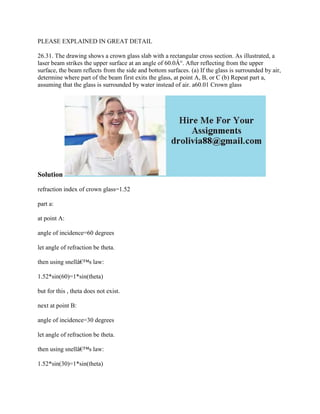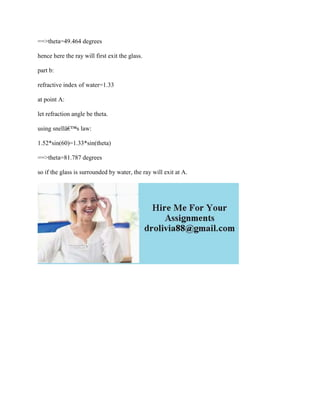Seu SlideShare está sendo baixado. ×

# PLEASE EXPLAINED IN GREAT DETAIL 26-31- The drawing shows a crown glas.docx

Anúncio
Anúncio
Anúncio
Anúncio
Anúncio
Anúncio
Anúncio
Anúncio
Anúncio
Anúncio
AnúncioCarregando em…3
×

1 de 2 Anúncio

# PLEASE EXPLAINED IN GREAT DETAIL 26-31- The drawing shows a crown glas.docx

26.31. The drawing shows a crown glass slab with a rectangular cross section. As illustrated, a laser beam strikes the upper surface at an angle of 60.0Â°. After reflecting from the upper surface, the beam reflects from the side and bottom surfaces. (a) If the glass is surrounded by air, determine where part of the beam first exits the glass, at point A, B, or C (b) Repeat part a, assuming that the glass is surrounded by water instead of air. a60.01 Crown glass
Solution
refraction index of crown glass=1.52
part a:
at point A:
angle of incidence=60 degrees
let angle of refraction be theta.
then using snellâ€™s law:
1.52*sin(60)=1*sin(theta)
but for this , theta does not exist.
next at point B:
angle of incidence=30 degrees
let angle of refraction be theta.
then using snellâ€™s law:
1.52*sin(30)=1*sin(theta)
==>theta=49.464 degrees
hence here the ray will first exit the glass.
part b:
refractive index of water=1.33
at point A:
let refraction angle be theta.
using snellâ€™s law:
1.52*sin(60)=1.33*sin(theta)
==>theta=81.787 degrees
so if the glass is surrounded by water, the ray will exit at A.
.

26.31. The drawing shows a crown glass slab with a rectangular cross section. As illustrated, a laser beam strikes the upper surface at an angle of 60.0Â°. After reflecting from the upper surface, the beam reflects from the side and bottom surfaces. (a) If the glass is surrounded by air, determine where part of the beam first exits the glass, at point A, B, or C (b) Repeat part a, assuming that the glass is surrounded by water instead of air. a60.01 Crown glass
Solution
refraction index of crown glass=1.52
part a:
at point A:
angle of incidence=60 degrees
let angle of refraction be theta.
then using snellâ€™s law:
1.52*sin(60)=1*sin(theta)
but for this , theta does not exist.
next at point B:
angle of incidence=30 degrees
let angle of refraction be theta.
then using snellâ€™s law:
1.52*sin(30)=1*sin(theta)
==>theta=49.464 degrees
hence here the ray will first exit the glass.
part b:
refractive index of water=1.33
at point A:
let refraction angle be theta.
using snellâ€™s law:
1.52*sin(60)=1.33*sin(theta)
==>theta=81.787 degrees
so if the glass is surrounded by water, the ray will exit at A.
.

Anúncio
Anúncio

### PLEASE EXPLAINED IN GREAT DETAIL 26-31- The drawing shows a crown glas.docx

1. 1. PLEASE EXPLAINED IN GREAT DETAIL 26.31. The drawing shows a crown glass slab with a rectangular cross section. As illustrated, a laser beam strikes the upper surface at an angle of 60.0Â°. After reflecting from the upper surface, the beam reflects from the side and bottom surfaces. (a) If the glass is surrounded by air, determine where part of the beam first exits the glass, at point A, B, or C (b) Repeat part a, assuming that the glass is surrounded by water instead of air. a60.01 Crown glass Solution refraction index of crown glass=1.52 part a: at point A: angle of incidence=60 degrees let angle of refraction be theta. then using snellâ€™s law: 1.52*sin(60)=1*sin(theta) but for this , theta does not exist. next at point B: angle of incidence=30 degrees let angle of refraction be theta. then using snellâ€™s law: 1.52*sin(30)=1*sin(theta)
2. 2. ==>theta=49.464 degrees hence here the ray will first exit the glass. part b: refractive index of water=1.33 at point A: let refraction angle be theta. using snellâ€™s law: 1.52*sin(60)=1.33*sin(theta) ==>theta=81.787 degrees so if the glass is surrounded by water, the ray will exit at A.